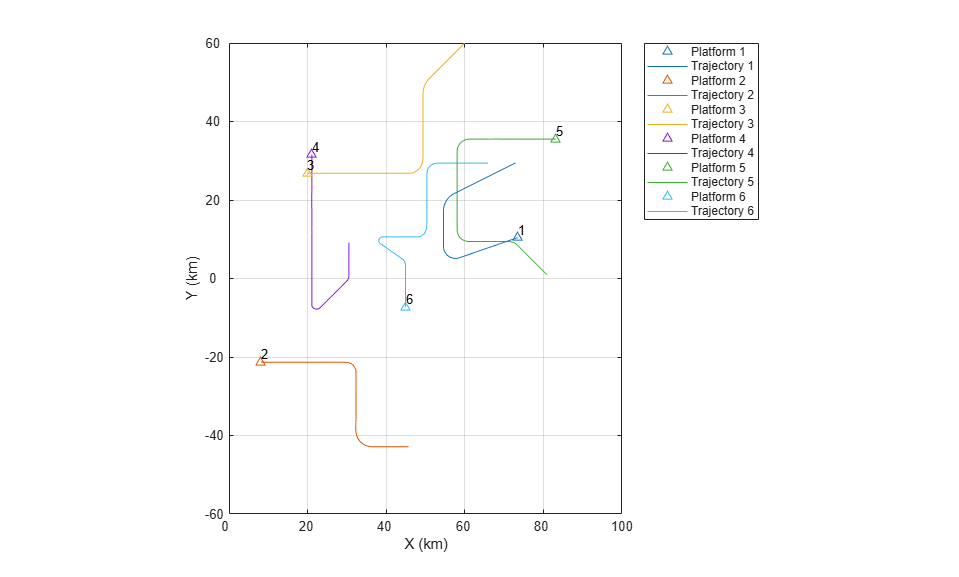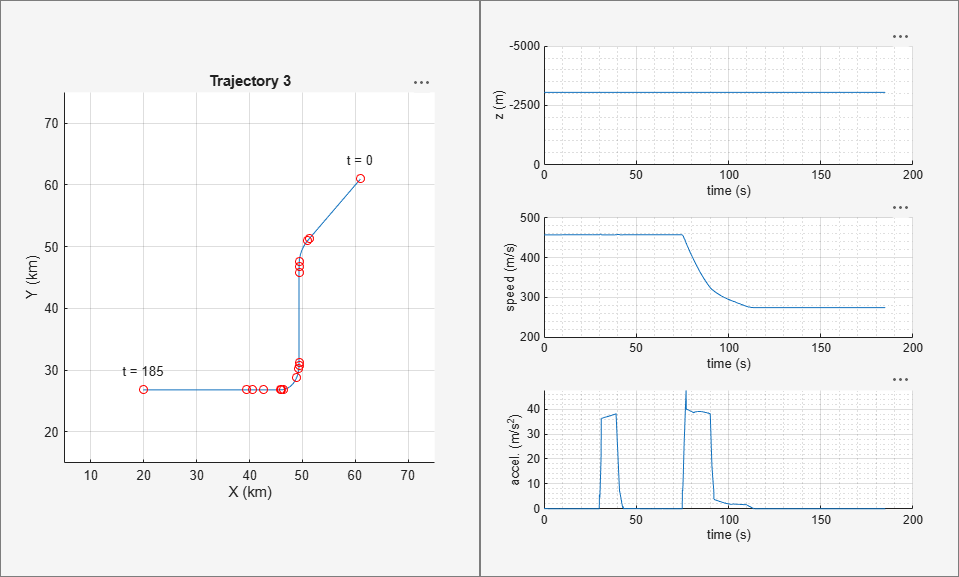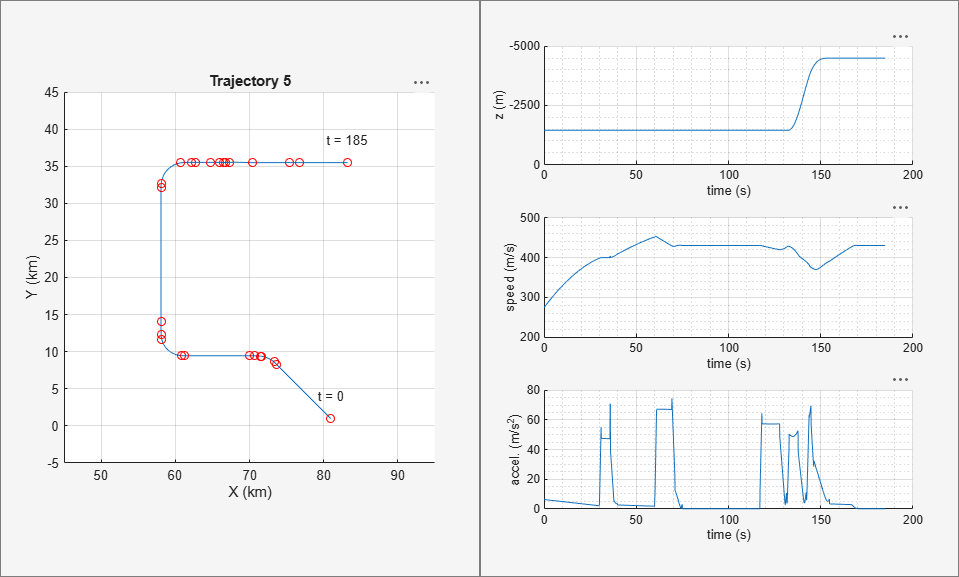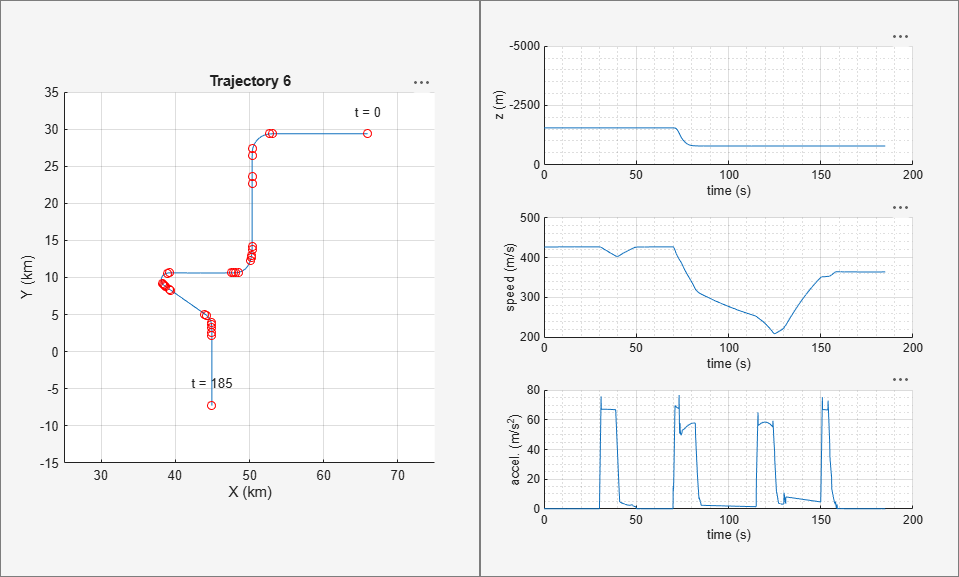# Benchmark Trajectories for Multi-Object Tracking

This example shows how to generate and visualize trajectories of multiple aircraft using `trackingScenario` and `waypointTrajectory`.

### Introduction

The six aircraft trajectories modeled in this example are described in . The aircraft fly in an arrangement intended to be received by a radar located at the origin.

### Choice of Interpolant

Conceptually speaking, a trajectory is a curve through space which an object travels as a function of time. To define the curve, you may think of a curve through space that passes through a set of points called waypoints connected by an interpolating function called an interpolant. An interpolant allows you to define the path between waypoints via a continuous function. Common interpolants are polynomial based (for example, piecewise linear or cubic splines). For a rapidly changing trajectory, more waypoints are required to keep the interpolated curve as close to the true curve as possible; however, we can reduce the number of required points by choosing interpolants carefully.

Many motion models used in track filters consist of "constant velocity," "constant turn," or "constant acceleration" profiles. To accommodate these motion models, the interpolant used in the `waypointTrajectory` object is based on a piecewise clothoid spline (horizontally) and a cubic spline (vertically). The curvature of a clothoid spline varies linearly with respect to distance traveled; this lets us model straight and constant turns with ease, having one extra degree of freedom to transition smoothly between straight and curved segments. Similarly, objects in the air experience the effects of gravity, following a parabolic (quadratic) path. Having a cubic spline to model vertical elevation allows us to model the path with a similar extra degree of freedom.

Once the physical path through space of an object is known (and set), the speed of the object as a function of distance traveled is determined via cubic Hermite interpolation. This is useful for modeling trajectories of objects that accelerate through turns or straight segments.

The benchmark trajectories we are using consist of straight, constant-g turns, and turns with acceleration.

### Waypoint Construction

The following file contains tables of waypoints and velocities (in units of meters and meters per second) that can be used to reconstruct six aircraft trajectories. Load it into MATLAB and examine the table containing the first trajectory.

```load('benchmarkTrajectoryTables.mat', 'trajTable'); trajTable{1} ```
```ans = 14x3 table Time Waypoints Velocities _____ _________________________ _____________________________ 0 72947 29474 -1258 -258.9 -129.69 0 60 57413 21695 -1258 -258.9 -129.66 0 62 56905 21417 -1258 -245.3 -153.89 0 78.1 54591 17566 -1258 -20.635 -288.86 0 80 54573 17016 -1258 -2.8042 -289.59 0 83 54571 16147 -1258 -0.061 -289.56 0 110 54571 8329 -1258 0 -289.56 0 112.7 54634 7551.5 -1258 58.979 -283.56 0 120 55718 5785.5 -1258 226.41 -180.59 0 129 58170 5172.8 -1258 284.74 52.88 0 132 59004 5413.9 -1258 274.26 93.05 0 137.8 60592 5962.2 -1258 273.62 94.76 0 147.8 63328 6909.9 -1258 273.62 94.76 0 185 73508 10435 -1258 273.62 94.76 0 ```

### Scenario Generation

The table contains a set of waypoints and velocities that the aircraft passes through at the corresponding time.

To use the control points, you can create a scenario with six platforms and assign a trajectory to each:

```scene = trackingScenario('UpdateRate',10); for n=1:6 plat = platform(scene); traj = trajTable{n}; plat.Trajectory = waypointTrajectory(traj.Waypoints, traj.Time, 'Velocities', traj.Velocities); end ```

### Trajectory Visualization

Once you have the scenario and plotter set up, you can set up a `theaterPlot` to create an animated view of the locations of the aircraft as time progresses.

```helperPlot = helperBenchmarkPlotter(numel(scene.Platforms)); while advance(scene) % extract the pose of each of the six aircraft poses = platformPoses(scene); % update the plot update(helperPlot, poses, scene.SimulationTime); end ```The trajectories plotted above are three-dimensional. You can rotate the plot so that the elevation of the trajectories is readily visible. You can use the `view` and `axis` commands to adjust the plot. Because the trajectories use a NED (north-east-down) coordinate system, elevation above ground has a negative z component.

```view(60,10); axis square grid minor set(gca,'ZDir','reverse'); ```### Trajectory 1

It may be instructive to view the control points used to generate the trajectories. The following figure shows the first trajectory, which is representative of a large aircraft.

The control points used to construct the path are plotted on the leftmost plot. Only a few waypoints are needed to mark the changes in curvature as the plane takes a constant turn.

The plots on the right show the altitude, magnitude of velocity (speed), and magnitude of acceleration, respectively. The speed stays nearly constant throughout despite the abrupt change in curvature. This is an advantage of using the clothoid interpolant.

```[time, position, velocity, acceleration] = cumulativeHistory(helperPlot); helperTrajectoryViewer(1, time, position, velocity, acceleration, trajTable); ```### Trajectory 2

The second trajectory, shown below, represents the trajectory of a small maneuverable aircraft. It consists of two turns, having several changes in acceleration immediately after the first turn and during the second turn. More waypoints are needed to adjust for these changes, however the rest of the trajectory requires fewer points.

```helperTrajectoryViewer(2, time, position, velocity, acceleration, trajTable); ```### Trajectory 3

The third trajectory, shown below is representative of a higher speed aircraft. It consists of two constant turns, where the aircraft decelerates midway throughout the second turn. You can see the control points that were used to mark the changes in velocity and acceleration in the x-y plot on the left.

```helperTrajectoryViewer(3, time, position, velocity, acceleration, trajTable); ```### Trajectory 4

The fourth trajectory, also representative of a higher speed aircraft, is shown below. It consists of two turns, where the aircraft accelerates and climbs to a higher altitude.

```helperTrajectoryViewer(4, time, position, velocity, acceleration, trajTable); ```### Trajectory 5

The fifth trajectory is representative of a maneuverable high-speed aircraft. It consists of three constant turns; however it accelerates considerably throughout the duration of the flight. After the third turn the aircraft ascends to a level flight.

```helperTrajectoryViewer(5, time, position, velocity, acceleration, trajTable); ```### Trajectory 6

The sixth trajectory is also representative of a maneuverable high-speed aircraft. It consists of four turns. After the second turn the aircraft decreases altitude and speed and enters the third turn. After the third turn it accelerates rapidly and enters the fourth turn, continuing with straight and level flight.

```helperTrajectoryViewer(6, time, position, velocity, acceleration, trajTable); ```### Summary

This example shows how to use `waypointTrajectory` and `trackingScenario` to create a multi-object tracking scenario. In this example you learned the concepts behind the interpolant used inside `waypointTrajectory` and were shown how a scenario could be reproduced with a small number of waypoints.

### Reference

1. W.D. Blair, G. A. Watson, T. Kirubarajan, Y. Bar-Shalom, "Benchmark for Radar Allocation and Tracking in ECM." Aerospace and Electronic Systems IEEE Trans on, vol. 34. no. 4. 1998

##### SupportGet trial now